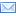#Difference Between Similar Terms and Objects

# Difference Between Yield and Interest RateYield vs Interest Rate

Do you know the difference between yield and interest rate? In order to start making smart investment decisions, and calculating your investment profits, you need to be able to tell the difference. To keep it as simple as possible, we can say that the yield is the profit, and the interest rate is why you made the profit.

To go a bit deeper, the interest rate is stated as a percentage. Whether you’re paying or receiving dividends, the interest rate is the percentage of money above the initial amount. If you take out a loan with a 3% interest rate, you will pay 3% more money than you borrowed. The same applies to profit. If you take out a CD with a 3% interest rate, you can expect to cash out the CD with the original deposit, plus an additional 3%.

The yield is how much you actually profited from the investment. While you can put the yield into terms of percentages, you can also do it simply in dollar amounts. If you bought an investment for \$10,000, with a 3% interest rate for one year, your yield would be about \$300. Of course, if you’re compounding your interest back into the investment, your yield increases. You can’t compound an interest rate.

Yield is determined through basic math. Take your initial investment, and simply divide it by the interest rate. This will give your basic term yield. Whether a term is 6 months, a year, or 12 years, you multiply your annual yield into the number of terms you’ll be allowing the investment to sit (if you’re not compounding), and you will derive your total yield. If you’re compounding, you’ll have to continually adjust the initial amount for each time the yield is compounded back into the investment. So, if you invested \$10,000 for three years, at a term of one year, your second term would be calculated by multiplying \$10,300 by the interest rate.

Summary:

1. Yield is the monetary profit.

2. Interest rate is how much additional monetary profit is produced per term.

3. Interest rate is bound in percentages.

4. Yield can be expressed in dollars or percentages.

### Search DifferenceBetween.net :

Custom Search

Help us improve. Rate this post!(4 votes, average: 2.75 out of 5)Loading...Email This Post : If you like this article or our site. Please spread the word. Share it with your friends/family.

1. You say

“Yield is determined through basic math. Take your initial investment, and simply divide it by the interest rate. This will give your basic term yield.”

In this example, would your basic term yield be 333,333.333… since \$10,000/0.03 = 333,333.333…?

Cause that doesn’t sound right.

• Sure they meant “multiply.”

Please note: comment moderation is enabled and may delay your comment. There is no need to resubmit your comment.

Articles on DifferenceBetween.net are general information, and are not intended to substitute for professional advice. The information is "AS IS", "WITH ALL FAULTS". User assumes all risk of use, damage, or injury. You agree that we have no liability for any damages.